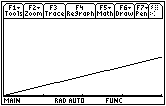# TI-89 Titanium Graphing Calculator LessonModule 16: The Fundamental Theorem of Calculus

## Lesson 16.2: Area Functions, A Visual Approach

### This lesson develops area functions using a visual approach by exploring the values of the areas under a curve when the right-hand endpoint changes. The visualization is displayed by graphing a definite integral in which the right end point is variable.

If the curve and the left-hand endpoint are given, an area function can be found for a right-hand endpoint that varies. It's necessary to distinguish between the independent variables for the curve function and for the area function. If x is used to denote the variable right-hand endpoint of the region, a second variable, t, should be used in the description of the curve function.

Letting x represent the right-hand endpoint, the area under the curve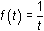and above the t-axis between t = 1 and t = x can be found by evaluating the definite integral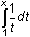.

For example, if x = 3 the area under the curve between x = 1 and x = 3 is about 1.09861, as illustrated and evaluated in the screens below.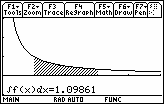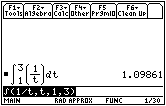16.2.1 Put your calculator in Approximate mode or just press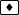to get the decimal values, and use the definite integral key to find the areas under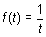between 1 and the following values of x. Record your results in the table.

 x 1.0 1.5 2.0 2.5 3.0 3.5 4.0 Area

16.2.2 Make a scatter plot of your results and find the logarithmic regression equation that best fits the data. Graph this equation together with the scatter plot.

Click on scatter plot or regression equation to review the associated keystrokes.

Based on this data, it appears that the area function, A(x), may be ln x.

Graphing Integrals

Graph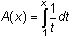together with the scatter plot.

• Disable or delete the regression equation in the Y=Editor
• Set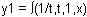and use the window [0.01, 4.5] x [-5, 2]
• Graph the equation y1

The graph will be slow to appear because the calculator has to compute a new definite integral for each point it plots. You should see the area functiondevelop point by point.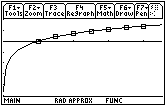Notice that the graph of A(x) appears to be the same as the graph of the ln x, the regression equation we found above.

16.2.3 Based on these graphs, predict the values of.

16.2.4 Describe how the Fundamental theorem applies.

Visualizing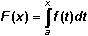Suppose that you don't know a formula for the curve function but you have its graph. You can determine the shape of the area function by analyzing the graph of the curve.

Below is the graph ofon the interval from 1 to x that was developed earlier.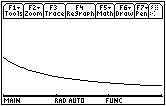Visualize the area under the graph of f being filled with thin rectangular sticks where the height of each stick is the y-value of the curve function. Each successive rectangular stick is shorter than the prior one. The Riemann sum is growing more slowly with each step as you move to the right because the area of each successive rectangle is less than the one before, as shown below.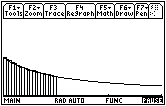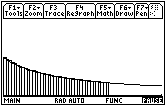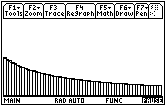Using the Fundamental Theorem

The Fundamental Theorem states that the derivative of the area function is the curve function. That means that the curve function values correspond to slopes of tangent lines of the area function.

Some of the characteristics of the area function that can be determined from the graph of the curve function are listed below.

1. A curve function's y-value at x is the slope of the tangent line of the area function at that x-value.
2. When the curve function is decreasing, the area function's slopes are decreasing and its graph is concave downward.
3. When the curve function is increasing, the area function's slopes are increasing and its graph is concave upward.

16.2.5 Examine the graph of f(x) between x = 0 and x = 4 shown below. Approximate the graph of the corresponding area function on the interval from 0 to 4.